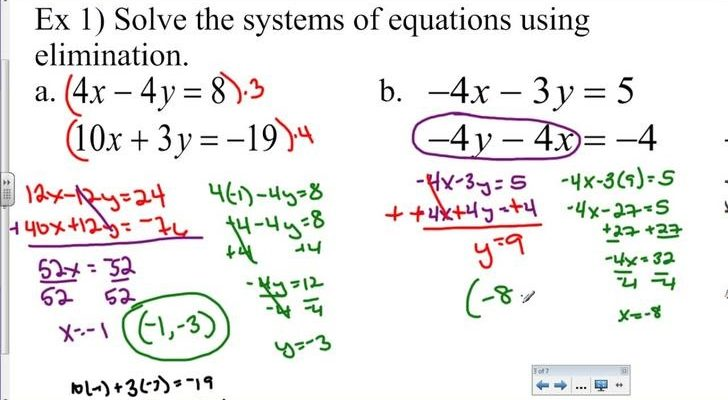BREAKING NEWS# Guide On Solving A System Of Equations With Substitution

Mathematics questions take different forms, this makes it quite difficult for students to know how to solve all kinds of questions given on a particular topic. This was a similar challenge I faced while trying to master how to solve any form of a system of equations question given to me.  But just like every other student, I wasn’t able to keep up with complex questions.

Being a student that always strive hard without relenting, I was able to figure out and understand a simple way of solving a system of equations and this has to do with mathematical substitution. This is what am about to share here in this article and am sure every student out there will find this solving a system of equations with substitution highly beneficial.

As a maths student, am expecting this method of solving systems of equations not to be completely new to you. nevertheless, I will simply this substitution method in the best possible way I can.

Having shared all that, let’s start by taking a quick look at what this method is all about and how it can be put to its best possible use when it comes to solving systems of equations.

How to use substitution method

Unlike other methods of solving a system of equations, substitution method simply make use of a process called substitution of variables. From what has been able to learn so far, all I do here is isolate one variable in either of the equations and use the isolated variable to find the value of the other variable.

This is a simple method of solving a system of equations and am sure anyone can use this for any question no matter how complex it seems on the outside. To elaborate all that is been explained above, I will solve an example to help further explain how to use substitution method to solve systems of equations.

An example of how to use substitution method

Let’s say we are given two equations, y = 2x and x + y = 24, using substitution method, the first thing to do is to isolate the value of one of the variables as explained earlier. In this case, the variable we will be isolating is x. Thus, the y=2x will be replaced in the second equation. The result of this will be x + 2x= 24. Summing them up, the equation changes to 3x=24.

The next step is to find the value of “x” and to do that, we have to divide both sides equally by 3. The answer we will be getting from this calculation is “8”. With this, we can then go ahead to find the value of “y” by substituting 8 which is the derived value of “x” in either of the equations above.

From this we will have x + y= 24, 8 + y=24. By adding “-8” to both sides, the value of y becomes 16.

By solving a system of equations with substitution, our value of y and x are 16 and 8 respectively.

Solving a system of equations with substitution are a big part of the math sections of the SAT. You can prepare for the SAT at Caddell Prep if you need further assistance on this topic.